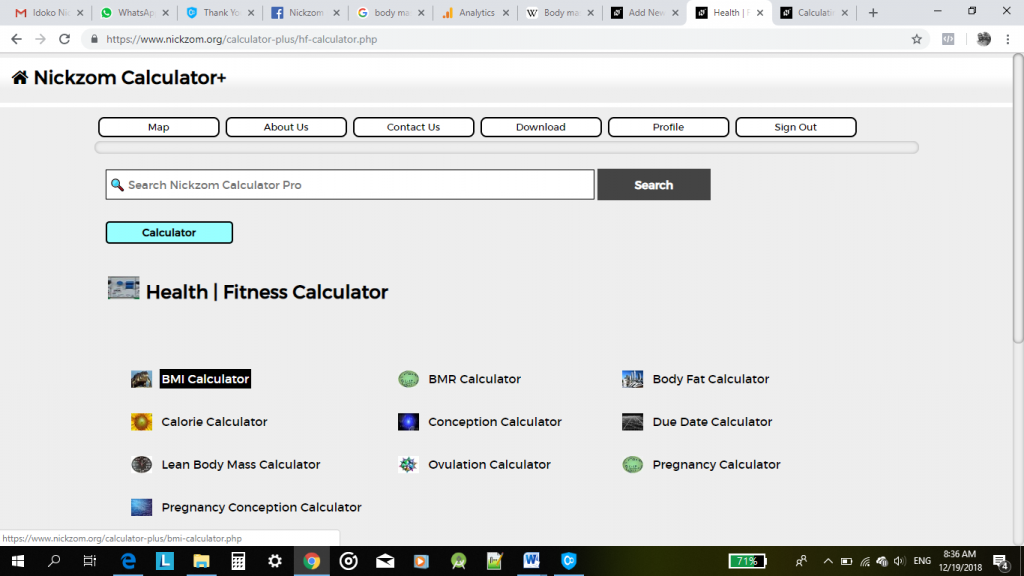## Nickzom Calculator Calculates Conception and Best Intercourse Dates

According to Wikipedia,

Conception, the beginning of pregnancy involving fertilisation (the fusion of gametes to produce an embryo) and implantation of the embryo onto the uterine wall.

Nickzom Calculator is capable of informing you on the conception date of your baby, due date of the baby and the most probable and possible conception. There are two major parameters that needs to be provided to obtain the required information.

• First Day of Your Last Period
• Average Length of Cycles

Nickzom Calculator provides 6 subsequent cycle dates on the following dates:

• Most Probable Conception Dates
• Best Intercourse Days
• Possible Conception Days
• Estimated Due Date

Nickzom Calculator is the very first calculator encyclopedia to come out from Nigeria. It is capable of solving over 50,000 calculations and presenting the steps (workings). Fortunately, Nickzom Calculator can compute conception and best intercourse dates.

## Nickzom Calculator Calculates Basal Metabolic Rate

According to Wikipedia,

Basal Metabolic Rate is a variant of standard metabolic rate measurement that excludes the temperature data, a practice that has led to problems in defining “standard” rates of metabolism for many mammals.

Basal Metabolic Rate is the rate of energy expenditure per unit time by endothermic animals at rest. It is reported in energy units per unit time ranging from watt (joule/second) to ml O2/min or joule per hour per kg body mass J/(h·kg). Proper measurement requires a strict set of criteria be met. These criteria include being in a physically and psychologically undisturbed state, in a thermally neutral environment, while in the post-absorptive state (i.e., not actively digesting food).

Nickzom Calculator is the very first calculator encyclopedia to come out from Nigeria. It is capable of solving over 50,000 calculations and presenting the steps (workings). Fortunately, Nickzom Calculator can solve for your body metabolic rate.

You can access Nickzom Calculator via any of these means:
Web – https://www.nickzom.org/calculator-plus
Apple (Paid) – https://itunes.apple.com/us/app/nickzom-calculator/id1331162702?mt=8

Once, you have access to Nickzom Calculator proceed to the Calculator Map, click on Health | Fitness Calculator under Calculator section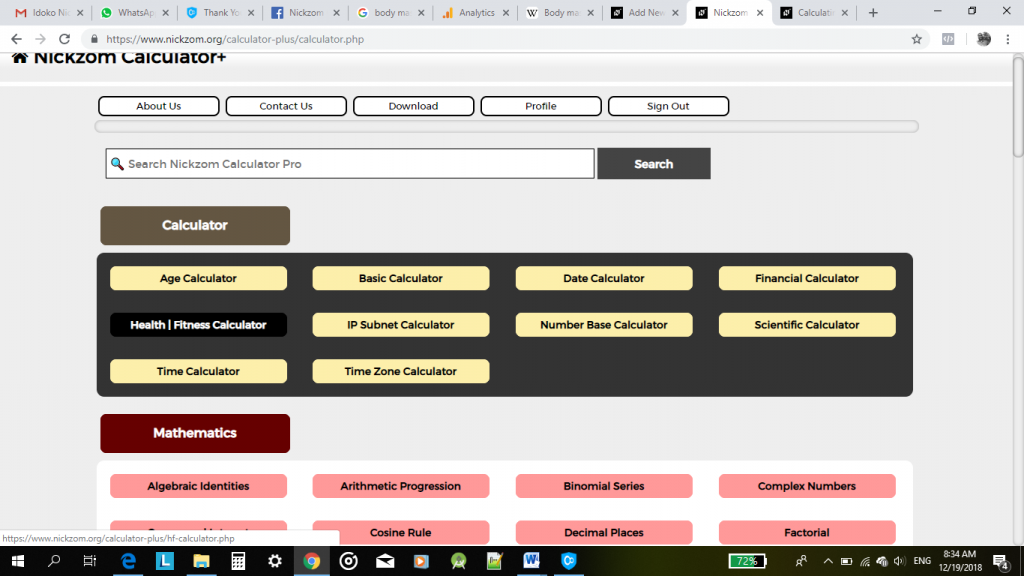Then, click on Basal Metabolic Rate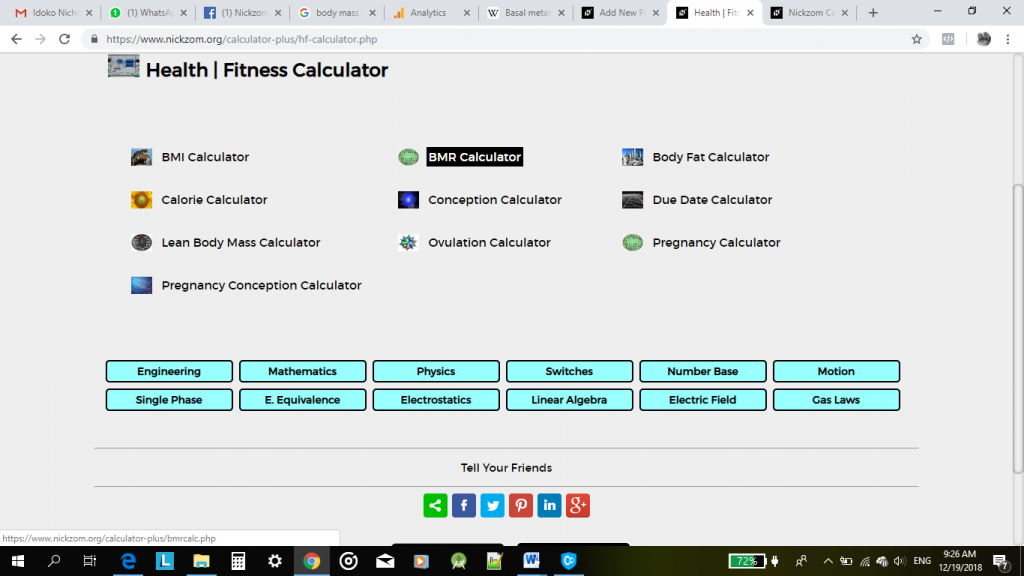Up next, comes a page or activity to enter the values for the respective parameters needed in computing the basal metabolic rate. These parameters according to Nickzom Calculator are:

• Age
• Gender
• Weight (in Kilograms)
• Height (in Centimeters)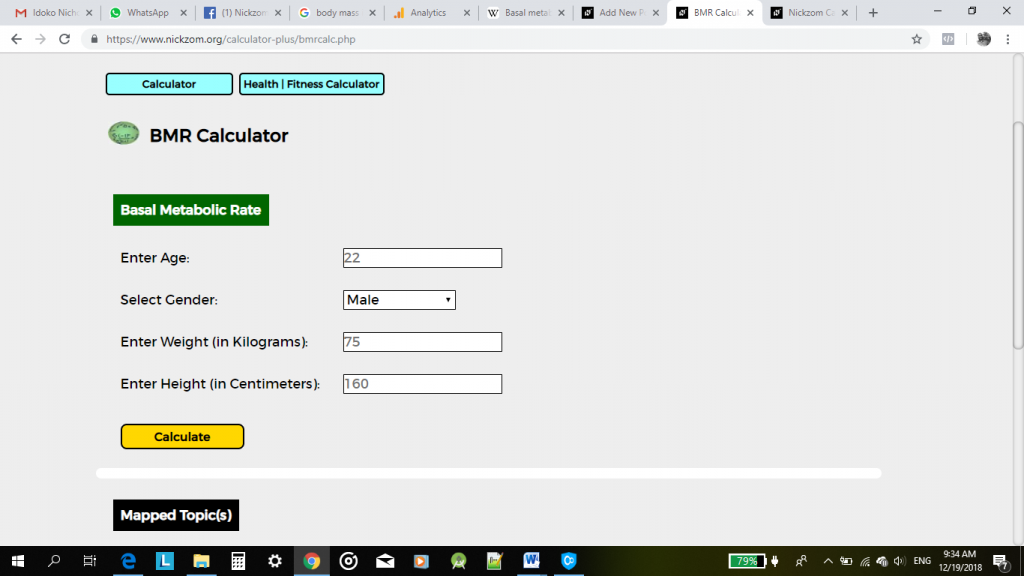For Example: I am 23 years old. I am a male. I weight 105 Kilograms and I am 182 centimeters tall.

The screenshot below displays the values entered appropriately.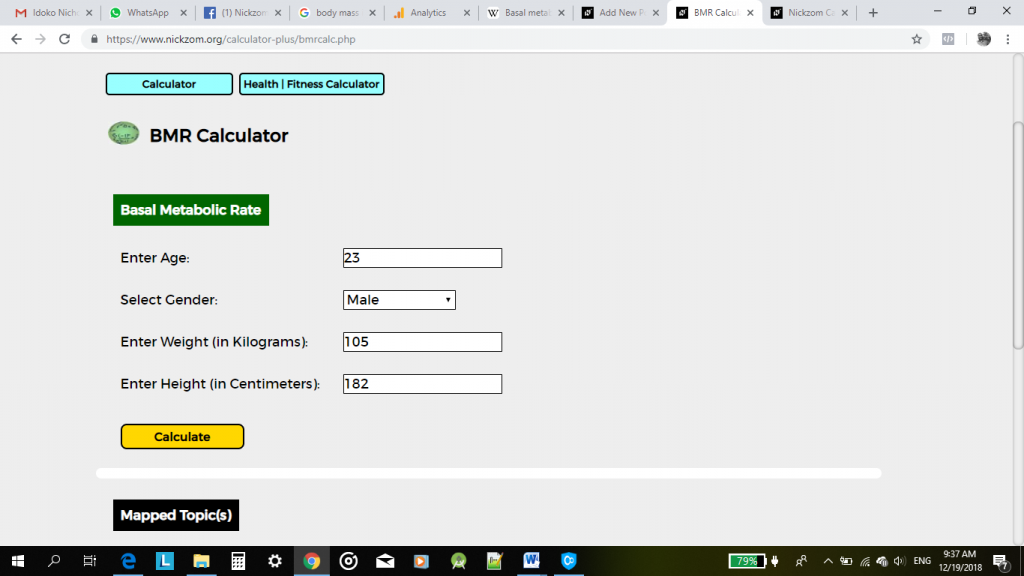Now, click on Calculate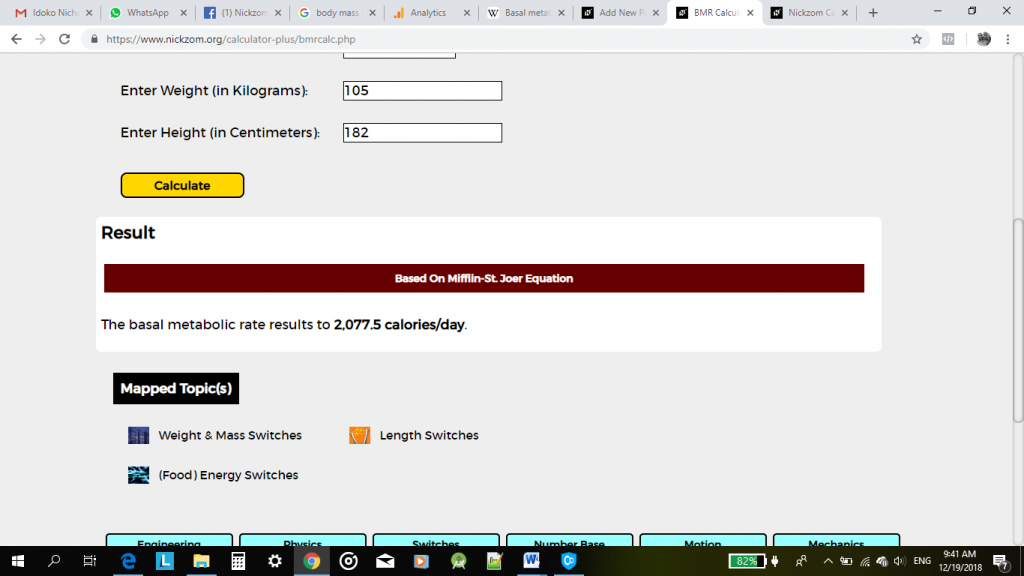Therefore, according to Mifflin-St. Joer equation, my basal metabolic rate is 2,077.5 calories per day.

## Nickzom Calculator Calculates Body Mass Index

According to Wikipedia,

The body mass index (BMI) or Quetelet index is a value derived from the mass (weight) and height of an individual. The BMI is defined as the body mass divided by the square of the body height, and is universally expressed in units of kg/m2, resulting from mass in kilograms and height in metres.

Nickzom Calculator is the very first calculator encyclopedia to come out from Nigeria. It is capable of solving over 50,000 calculations and presenting the steps (workings). Fortunately, Nickzom Calculator can solve for your body mass index.

You can access Nickzom Calculator via any of these means:
Web – https://www.nickzom.org/calculator-plus